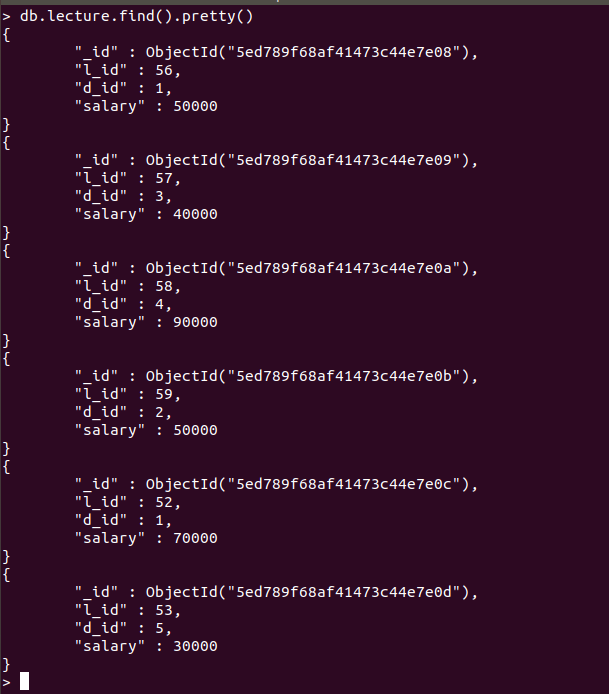# How to check if the PyMongo Cursor is Empty?

• Last Updated : 10 Jul, 2020

MongoDB is an open source NOSQL database, and is implemented in C++. It is a document oriented database implementation that stores data in structures called Collections (group of MongoDB documents). PyMongo is a famous open source library that is used for embedded MongoDB queries. PyMongo is widely used for interacting with a MongoDB database as python is powerful language for data analysis and data science.

## Check if the Cursor object is empty or not?

When a given collection is queried using pymongo using the .find() method, the return value is an object of type PyMongo.cursor.Cursor Class and it contains the documents matching the query. PyMongo cursor would be empty in the case the query made returns no result. If you want to get the individual documents you need to use the .find_one() method. To check is the cursor object is empty or not several approaches can be followed –

Approach 1: The cursor returned is an iterable, thus we can convert it into a list. If the length of the list is zero (i.e. List is empty), this implies the cursor is empty as well.

Sample Database:Example:

## Python3

 `import` `pymongo ` ` `  `connection ``=` `pymongo.MongoClient() ` `db ``=` `connection.GFG ` `col ``=` `db.lecture ` ` `  `# This is a cursor instance ` `cur ``=` `col.find()    ` ` `  `results ``=` `list``(cur) ` ` `  `# Checking the cursor is empty ` `# or not ` `if` `len``(results)``=``=``0``: ` `    ``print``(``"Empty Cursor"``) ` `else``: ` `    ``print``(``"Cursor is Not Empty"``) ` `    ``print``(``"Do Stuff Here"``) `

Output:

```Cursor is Not Empty
Do Stuff Here```

Approach 2: Another way is to use the .count() method that returns the number of matching documents for the query. if the return value for .count() is 0, then the cursor is empty

Example:

## Python3

 `import` `pymongo ` ` `  ` `  `connection ``=` `pymongo.MongoClient() ` `db ``=` `connection.GFG ` `col ``=` `db.lecture ` ` `  `# This is a cursor instance ` `cur ``=` `col.find() ` ` `  `if` `cur.count()``=``=``0``: ` `    ``print``(``"Empty Cursor"``) ` `else``: ` `    ``print``(``"Cursor is Not Empty"``) ` `    ``print``(``"Do Stuff Here"``)`

Output:

```Cursor is Not Empty
Do Stuff Here```

My Personal Notes arrow_drop_up
Recommended Articles
Page :